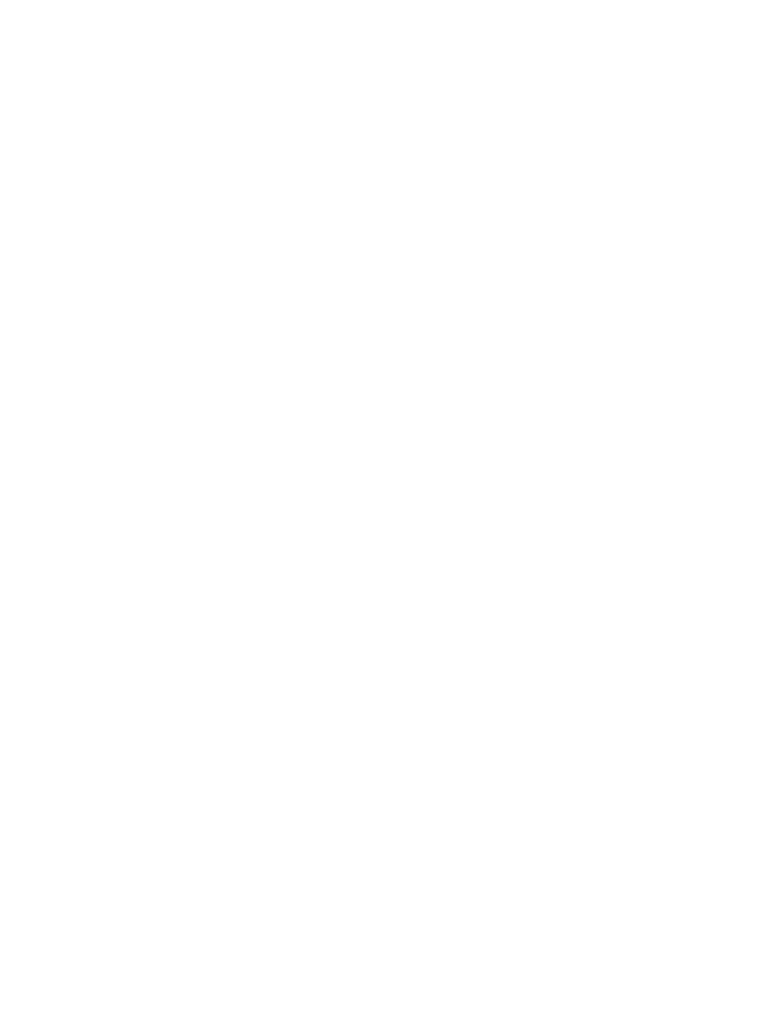Textbook Notes (270,000)
CA (160,000)
York (10,000)
Chapter 3

Department
Course Code
Professor
Natalie Guriel
Chapter
3

This preview shows half of the first page. to view the full 1 pages of the document.Chapter 3
18 bits to represent a little more than 5 decimals
Approximately 3.3 bits are required to represent a little more
than 5 decimal digits
ob/c 2^3.3=10
since binary multiplication table is so simple, multiplication can
be implemented in a computer fairly easily.
Shifting a binary number one position to the left has the e%ect of
doubling its value
In general, shifting a number in any base left one digit multiplies
its value by the base, and, conversely, shifting a number right
one digit divides its value by the base
Each hexadecimal number represents exactly 4 binary bits
Decimal speci+cally implies base 10
oTherefore use number point (binary point, hexadecimal
point, etc)